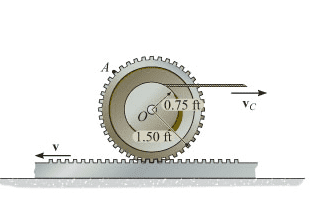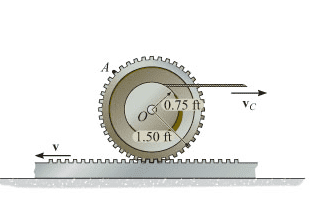# Instantaneous Center

## Homework Statement

Determine the angular velocity of the gear at the instant shown. Set v = 9ft/s and vc = 6ft/s. Assume counterclockwise is positive.v = w x rIC

## The Attempt at a Solution

I tried solving this many times using va = v0 + (ω x ra0 ) and vb=v0 + (ω x rb0 ) but kept getting the wrong answer.
Then I ended up solving for the instantaneous center using similar triangles and used v = ωxrIC.

How do I know when to use an instantaneous center in my problem solving?

mfb
Mentor
I tried solving this many times using va = v0 + (ω x ra0 ) and vb=v0 + (ω x rb0 ) but kept getting the wrong answer.
Then the problem is somewhere in the steps you did not show.
Just a guess: Did you take the signs into account properly?

## Homework Statement

Determine the angular velocity of the gear at the instant shown. Set v = 9ft/s and vc = 6ft/s. Assume counterclockwise is positive.v = w x rIC

## The Attempt at a Solution

I tried solving this many times using va = v0 + (ω x ra0 ) and vb=v0 + (ω x rb0 ) but kept getting the wrong answer.
Then I ended up solving for the instantaneous center using similar triangles and used v = ωxrIC.

How do I know when to use an instantaneous center in my problem solving?
Instantaneous centre method can be used in any problem.
But both methods give the same result. Maybe there's some problem with the signs like mfb said.

Thank you for helping! Sorry there was such a delay in my response. Yes it ended up being just a calculation error when mixing up the signs!